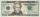Three friends

Cuba, Matthew and their friend Adam found a brigade during their weekend weekends because they wanted to make a joint trip to the Alps that they planned for the spring break.

Cuba enjoyed the skiing trip very much, so he was not lazy to get up and went to the brigade early in the morning. Thanks to this, he managed to earn 11,000 crowns, but Matěj instead of getting up early morning, he earned only one-third of the arithmetic average of the earnings of Adam and Cuba.
All three boys made up three times more than Adam himself. Make Adam's earnings a. Create an equation that lets you calculate how much money Adam has earned.

Also, to make the task complete, you also determine how much Matthew earned.

Result

a =  8800
k =  11000
m =  6600

Solution:

k = 11000
m = (a+k)/3
a+k+m = 3a

k = 11000
a+k-3m = 0
2a-k-m = 0

a = 8800
k = 11000
m = 6600

Calculated by our linear equations calculator.

Leave us a comment of this math problem and its solution (i.e. if it is still somewhat unclear...):Be the first to comment!To solve this verbal math problem are needed these knowledge from mathematics:

Looking for help with calculating arithmetic mean? Looking for a statistical calculator? Need help calculate sum, simplify or multiply fractions? Try our fraction calculator. Do you have a linear equation or system of equations and looking for its solution? Or do you have quadratic equation?

Next similar math problems:

1. Three unknownsSolve the system of linear equations with three unknowns: A + B + C = 14 B - A - C = 4 2A - B + C = 0
2. Three workshopsThere are 2743 people working in three workshops. In the second workshop works 140 people more than in the first and in third works 4.2 times more than the second one. How many people work in each workshop?
3. Boys and money270 USD boys divided so that Peter got three times more than Paul and Ivan has 120 USD more than than Paul. How much each received?
4. Theorem proveWe want to prove the sentence: If the natural number n is divisible by six, then n is divisible by three. From what assumption we started?
5. A fishermanA fisherman buys carnivores to fish. He could buy either 6 larvae and 4 worms for \$ 132 or 4 larvae and 7 worms per \$ 127. What is the price of larvae and worms? Argue the answer.
6. LegsCancer has 5 pairs of legs. The insect has 6 legs. 60 animals have a total of 500 legs. How much more are cancers than insects?
7. SavingsPaul has a by half greater savings than half Stanley, but the same savings as Radek. Staney save 120 CZK less than Radek. What savings have 3 boys together?
8. ChildrenThe group has 42 children. There are 4 more boys than girls. How many boys and girls are in the group?
9. Median and modusRadka made 50 throws with a dice. The table saw fit individual dice's wall frequency: Wall Number: 1 2 3 4 5 6 frequency: 8 7 5 11 6 13 Calculate the modus and median of the wall numbers that Radka fell.
10. Linsys2Solve two equations with two unknowns: 400x+120y=147.2 350x+200y=144
11. Nine booksNine books are to be bought by a student. Art books cost \$6.00 each and biology books cost \$6.50 each . If the total amount spent was \$56.00, how many of each book was bought?
12. 75th percentile (quartille Q3)Find 75th percentile for 30,42,42,46,46,46,50,50,54
13. Ball gameRichard, Denis and Denise together scored 932 goals. Denis scored 4 goals over Denise but Denis scored 24 goals less than Richard. Determine the number of goals for each player.
14. AliceAlice spent 5/11 of her money on a back pack. She has \$42 dollars left. How much was her back pack?
15. A candleA candle shop sells scented candles for \$16 each and unscented candles for \$10 each. The shop sells 28 candles today and makes \$400. a. Write a system of linear equations that represents the situation. b. Solve the system to answer the questions: How m
16. 925 USDFour classmates saved an annual total 925 USD. The second save twice as the first, third 35 USD more than the second and fourth 10 USD less than the first. How USD save each of them?
17. Elimination methodSolve system of linear equations by elimination method: 5/2x + 3/5y= 4/15 1/2x + 2/5y= 2/15